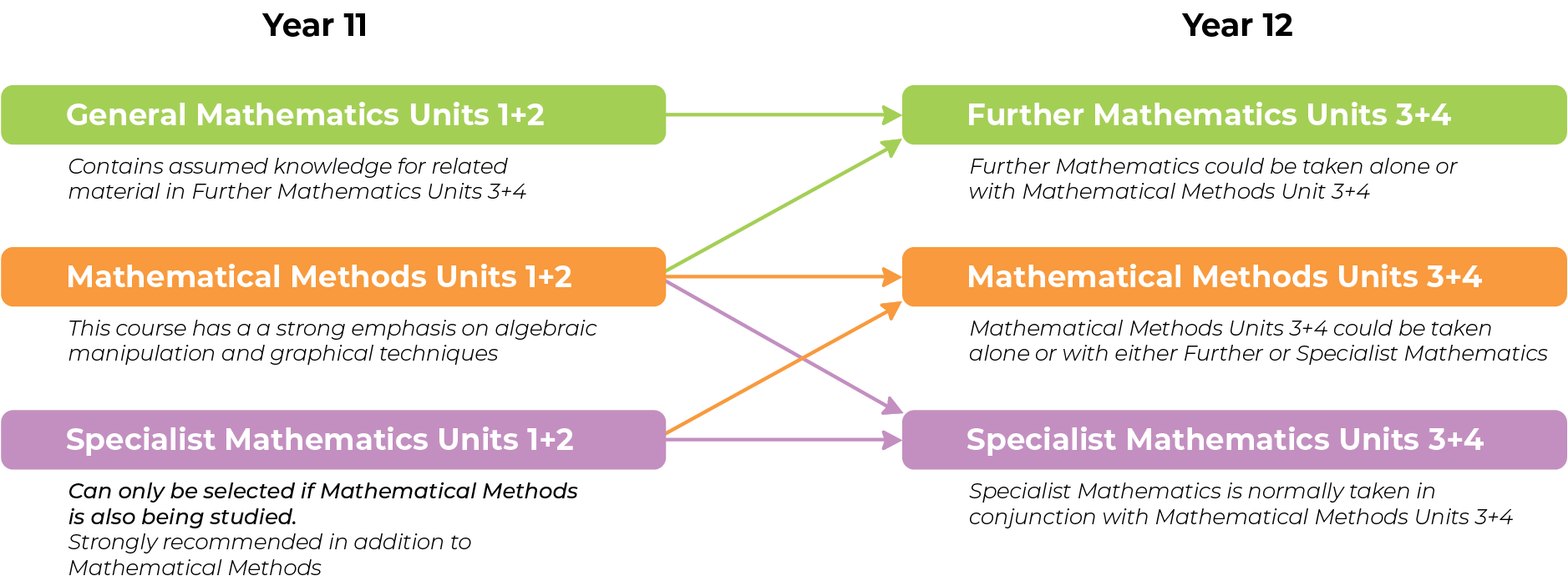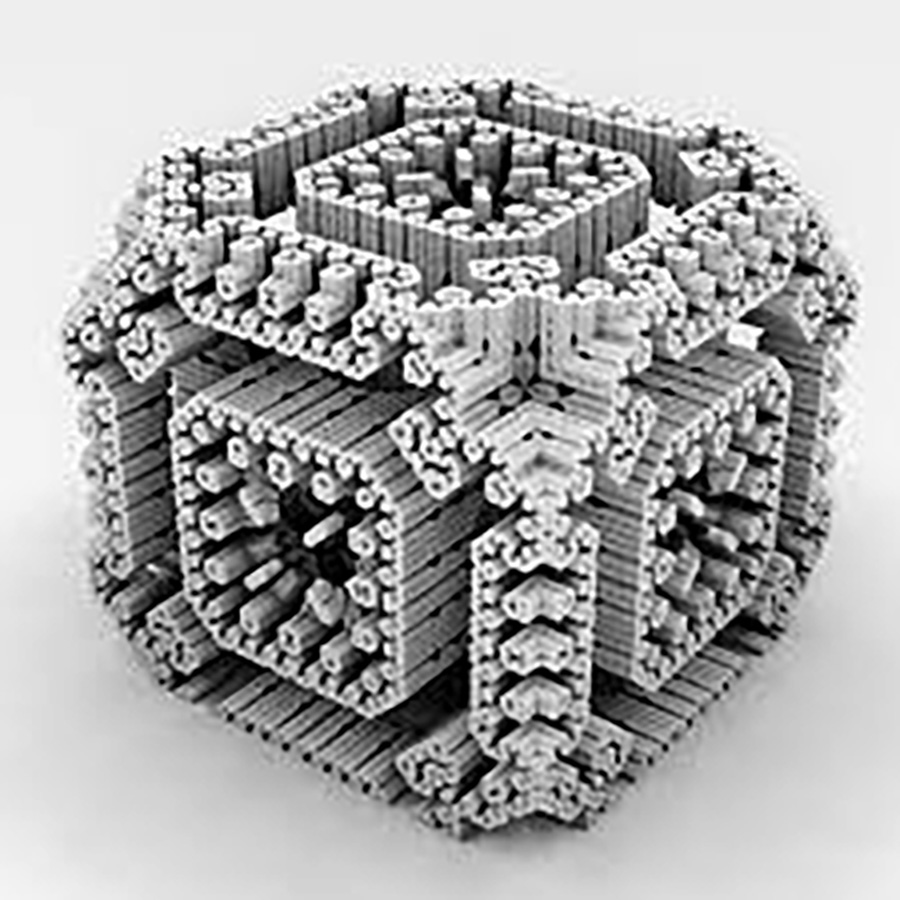# Mathematics Pathways# Foundation Mathematics#### Overview

Foundation Mathematics is recommended for Year 10 students who are intending to enrol in the VCAL stream in Year 11 or a Year 11 student who is not enrolled in Year 11 General Mathematics or year 11 Mathematical Methods.

This course by itself is not an adequate preparation for any further studies of VCE Mathematics. In this subject there is a strong emphasis on the use of Mathematics in practical contexts encountered in everyday life.

#### Areas of Study

The areas for study in both units are

• Space, Shape and Design
• Patterns and Number
• Data
• Measurement

#### Assessment

Assessment will include Investigations, assignments and projects.

There will be skill tests which will use a range of technologies where appropriate and skill tests without calculators.

# General Mathematics Unit 1+2#### Overview

This study is an excellent pathway and preparation for students wishing to go on to Units 3+4 Further Mathematics in Year 12.

On completion of these units the student should be able to:

• Define and explain key concepts as specified in the content from the areas of study and use this knowledge to solve routine application problems.
• Select and apply mathematical facts, concepts, models and techniques from the topics covered in the unit to investigate and analyse extended application problems in a range of contexts.
• Use a CAS calculator to develop mathematical ideas, produce results and carry out analysis in situations requiring problem-solving, modelling or investigative techniques or approaches

#### Unit Prerequisites

Students are expected to have completed Year 10 mathematics.

#### Areas of Study

There are six areas of study:

1. Algebra and Structure
2. Arithmetic and Number
3. Discrete Mathematics
4. Geometry
5. Measurement and Trigonometry
6. Graphs of linear and non-linear relations

#### Unit Assessment

Assessment tasks will be drawn from:

• assignments
• tests
• summary or review notes
• mathematical investigations

#### Pathways

General Mathematics Units 1 & 2 allows students to study Further Mathematics Units 3 & 4. A study of Mathematics is often a pre-requisite or an advantage to study beyond secondary school.

# Specialist Mathematics Unit 1+2#### Overview

On completion of these units the student should be able to:

• Define and explain key concepts as specified in the areas of study and apply a range of related mathematical routines and procedures
• Apply mathematical processes in non-routine contexts and analyse and discuss these applications of mathematics
• Use a CAS calculator to produce results and carry out analysis in situations requiring problem solving, modelling or investigative techniques or approaches

#### Unit Prerequisites

Specialist Mathematics may only be taken in conjunction with Mathematical Methods Units 1 & 2.

It is strongly advised that students who are thinking of choosing Specialist Mathematics Units 3 + 4 complete the sequence of Specialist Unit 1 + 2.

#### Areas of Study

• Algebra and Structure
• Arithmetic and Number
• Discrete Mathematics
• Geometry, Measurement and Trigonometry
• Graphs of linear and non-linear relations
• Statistics

#### Unit Assessment

Assessment tasks will be drawn from

• assignments
• tests
• summary or review notes
• mathematical investigations

#### Pathways

Specialist Mathematics Units 1 & 2 provides a comprehensive background for Mathematical Methods Units 3 & 4 and Specialist Mathematics Units 3 & 4. These subjects are strongly recommended for students hoping to enter the areas of Science Engineering, Applied Mathematics or Statistics.

# Mathematical Methods Unit 1+2#### Overview

On completion of these units the student should be able to:

• Define and explain key concepts as specified in the areas of study and apply a range of related mathematical routines and procedures
• Apply mathematical processes in non-routine contexts and analyse and discuss these applications of mathematics
• Use a CAS calculator to produce results and carry out analysis in situations requiring problem solving, modelling or investigative techniques or approaches.

#### Unit Prerequisites

All students must have completed Year 10 Mathematics to a satisfactory standard. it is strongly advised that they have completed their year 10 studies in Year 10 Maths A. Mathematical methods is a challenging subject and a strong ability and/ or determination are vital to taking on this challenge.

#### Areas of Study

• Functions and graphs
• Algebra
• Calculus
• Probability and Statistics

#### Unit Assessment

Assessment tasks will be drawn from

• assignments
• tests
• summary or review notes
• mathematical investigations

#### Pathways

Mathematical Methods Units 1 & 2 allows students to study Mathematical Methods Units 3 & 4 and Specialist Mathematics Units 3 & 4.
Other subjects that complement Mathematical Methods are: Specialist Mathematics, Chemistry, Physics, Algorithmics.

# Mathematical Methods Unit 3+4#### Overview

• Functions and Graphs
• Algebra
• Calculus
• Probability and Statistics

#### Unit Prerequisites

Students undertaking Unit 3 and 4 of Mathematical Methods are expected to have confidence in their abilities and .a strong understanding of Unit1 & 2 Mathematical Methods.

#### Assessment

• School assessed coursework (Application Task and two Modelling/Problem Solving Tasks) contributes 17 per cent for each of Units 3 and 4
• Units 3 and 4 are also assessed by two end of year examinations which contribute 66 per cent to the final assessment

#### Pathways

Other subjects that are often seen to complement Mathematical Methods are: Specialist Mathematics, Physics, Chemistry.

# Further Mathematics Unit 3+4#### Overview

On completion of these units the student should be able to:

• Define and explain key concepts as specified in the areas of study and use this knowledge to apply a range of related mathematical techniques and models in routine contexts
• Select and apply mathematical concepts, models and techniques in a range of contexts of increasing complexity
• Use a CAS calculator to produce results and carry out analysis in situations requiring problem-solving, modelling or investigative techniques or approaches.

#### Unit Prerequisites

This subject may be selected by students who have undertaken either General Mathematics 1 & 2 and or Mathematical Methods 1 & 2.

Highly able students who successfully undertake Mathematics A in Year 10 may apply to accelerate into Further Mathematics Units 3 & 4 in Year 11. If acceleration is approved, the student must also select Maths Methods Units 1 & 2.

#### Areas of Study

• Core: Data analysis
• Core: Recursion and financial modelling
• Selection of 2 out of 4 modules: Matrices, Networks and decision mathematics, Geometry and measurement, Graphs and relations

#### Unit Assessment

• School assessed coursework (Application Task and three Modelling/Problem Solving Tasks) for Unit 3 contributes 20 per cent and for Unit 4 contributes 14 per cent to the final assessment
• Units 3 & 4 are also assessed by two end of year examinations which contribute 66 per cent in total to the final assessment.